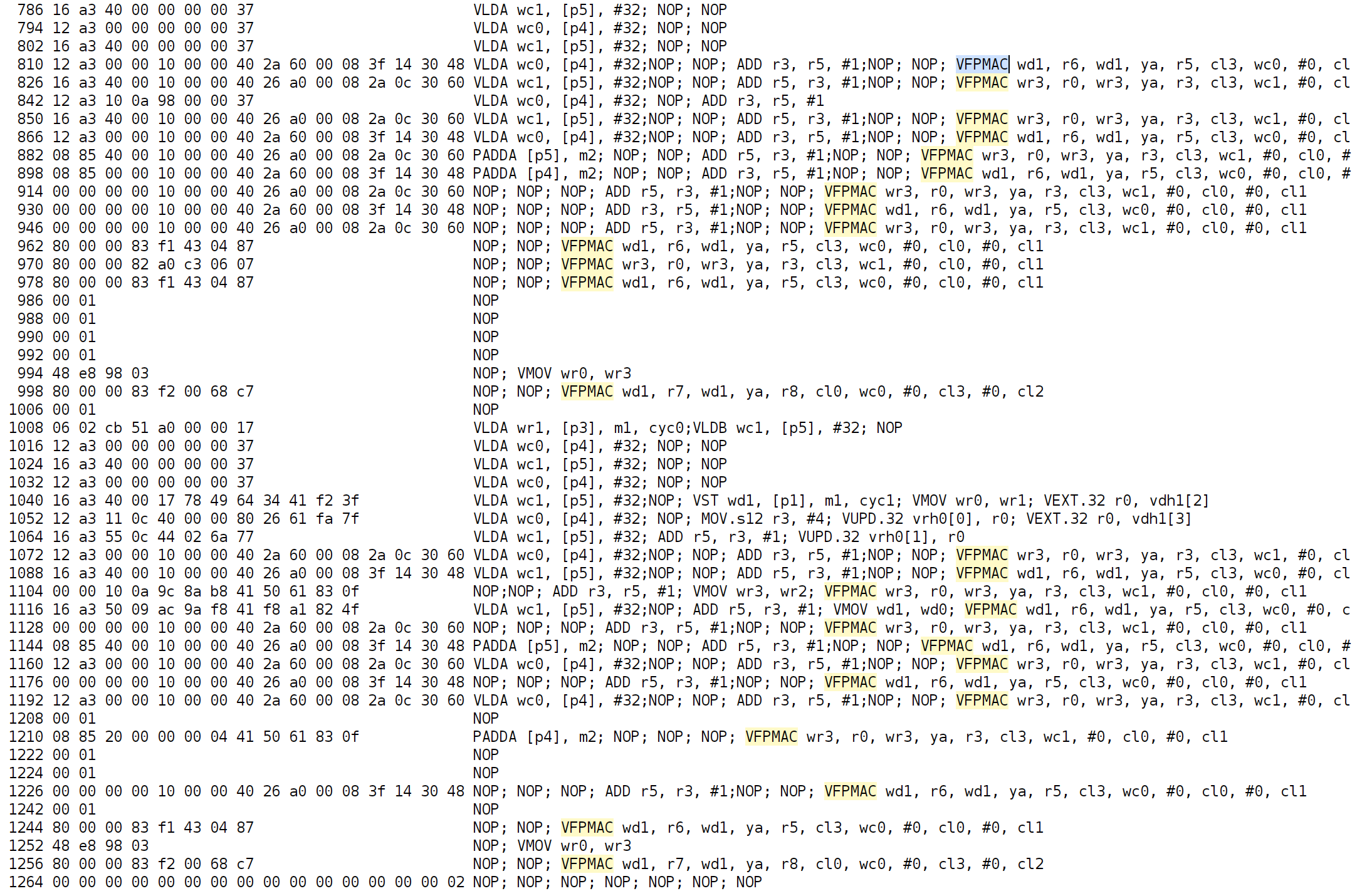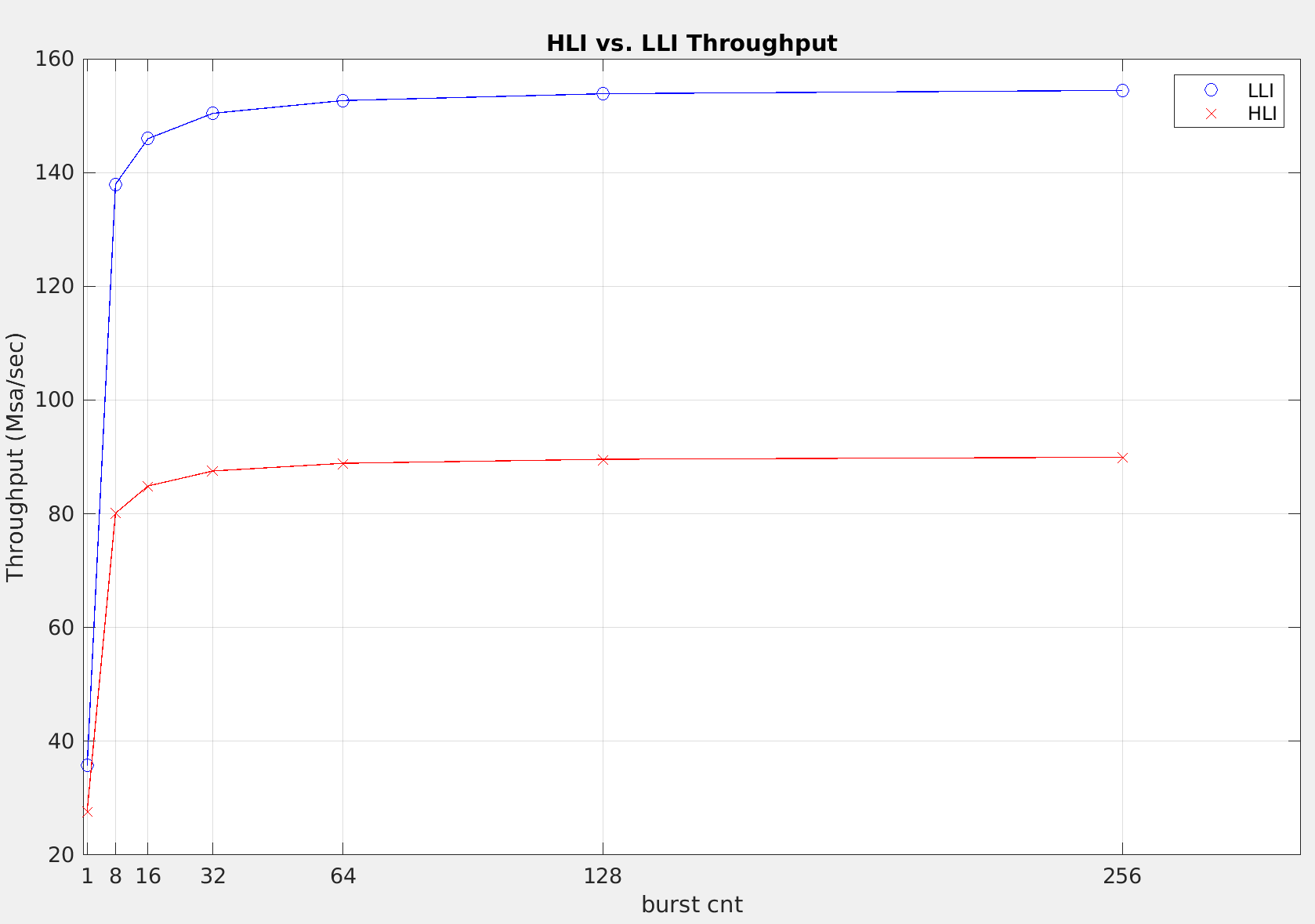# Kernel Code (LLI) - 2022.2 English

## Vitis Tutorials: AI Engine Development

Document ID
XD100
Release Date
2022-12-01
Version
2022.2 English
```#include <aie_api/aie_adf.hpp>

#include "kernel.hpp"

template<unsigned id>
void SecondOrderSection(
input_window_float *idata,
output_window_float *odata,iteration
const float (&C_e),			// run-time parameter: SIMD matrix of coefficients (even columns)
const float (&C_o)			// run-time parameter: SIMD matrix of coefficients (odd columns)
) {

static v8float state_reg = null_v8float();

for (auto i = 0; i < burst_cnt; i++) {

v16float xreg = concat(state_reg, xreg_hi);

v8float acc_e = null_v8float();
v8float acc_o = null_v8float();

v8float *ptr_coeff_e = (v8float *)(&C_e);
v8float *ptr_coeff_o = (v8float *)(&C_o);

for (auto j = 0; j < 6; j++)
chess_flatten_loop
{

v8float coeff_e = *ptr_coeff_e++;
acc_e = fpmac(acc_e, xreg, (2 * j + 4), 0, coeff_e, 0, 0x76543210);

v8float coeff_o = *ptr_coeff_o++;
acc_o = fpmac(acc_o, xreg, (2 * j + 5), 0, coeff_o, 0, 0x76543210);

} // end for (auto j = 0; j < 6; j++)

window_writeincr(odata, acc_o);

// update states
state_reg = xreg_hi;
state_reg = upd_elem(state_reg, 4, ext_elem(acc_o, 6));
state_reg = upd_elem(state_reg, 5, ext_elem(acc_o, 7));

} // end for (auto i = 0; i < burst_cnt; i++)

} // end SecondOrderSection()
```

Note the use of the `chess_flatten_loop` pragma. This pragma unrolls the loop completely, eliminating the loop construct. Documentation on compiler pragmas may be found in the AI Engine Lounge.

Note: In the code provided, selecting between API and LLI is performed by defining or commenting out `USE_API` on line 25 of `kernel.hpp`.

The generated assembly code is shown below.Note the “tighter” spacing between `VFPMAC`s. Also note that the `SecondOrderSection<1>` function has been “absorbed” into the main function, and the there are two unrolled matrix-vector multiplication loops, effectively halving the number of iterations of the outer loop.

The measured throughput is shown below (see `lli_thruput.xlsx`).

IIR Throughput (with LLI) | | | | | | | | | |—————————|——-|——-|——-|——-|——-|——-|——-| |burst_cnt |1 |8 |16 |32 |64 |128 |256 | |num_samples |8 |64 |128 |256 |512 |1024 |2048 | |num_cycles (LLI) |224 |464 |877 |1702 |3354 |6656 |13261 | |LLI Throughput (Msa/sec)) |35.71 |137.93 |145.95 |150.41 |152.65 |153.85 |154.44 |

*clk_freq: 1GHz

Comparing the API and LLI throughputs:• LLI provides a better throughput than API for the same `burst_cnt`

• The throughput “saturates” at around `burst_cnt` = 64